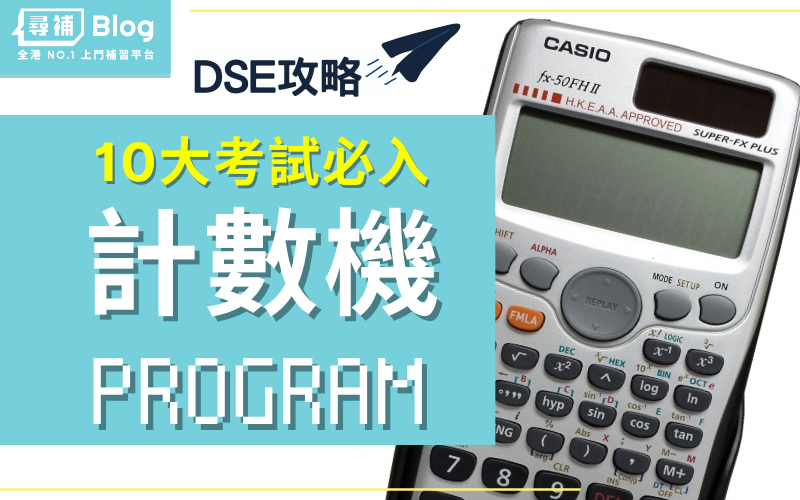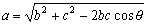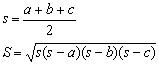【計數機Program】MC必備！DSE數學CASIO計算機程式攻略

## 10大DSE必入計數機program

### 輸入Program小貼士：

1. 程式中綠色部份可以不輸入。
2. Bytes的數字是讓大家可以檢查一下自己輸入時有沒有打多或打少了符號
3. 如果按EXE後，計算機右上角出現R<=>I，表示為複數解
4. 輸入後建議先用例題試一次，否則到了考DSE時才發現用不到／ERROR，就後悔不已了！
5. 每部計數機的記憶有限，不能夠輸入所有Program（也聽聞有同學會買多過一部計數機），所以要慎選，更要記得如何打開特定的計數機Program

👇🏻 先看圖，下面有詳細說明👇🏻

## 1. 一元二次方程(I) (Quadratic equations I)

?→A: ?→B: ?→M: – B┘(2A→C: AC2M-:

C+√( -M┘A→A◢ 2C – Ans→B

## 2.  一元三次方程(II) (Cubic Equations II)

?→A: ?→B: ?→C: ?→M: – B┘(3A→B: BCM+:

B3 – M┘(2A→M: B2 – C┘(3A→C: M2 – C3:

If Ans>0: Then √( AnsM+: B + 3√( M ) + 3√( M – 2Ans◢ Lbl IfEnd:

2√( C→C: C => 3-1 cos-1( 8M÷C3→M: B + C cos(M◢

B – C cos( M + 60o◢ B – C cos( M – 60o

?→A: ?→B: ?→C: ?→M: – B┘(3A→B: BCM+:

B3 – M┘(2A→M: B2 – C┘(3A: √(M2 – Ans3M+:

If Ans=Conjg( Ans: Then 3√( M – 2Ans→C: 3√( M→M:

Else 3√( Abs( M ) )∠( 3-1arg( M→M: Conjg( M→C: IfEnd:

M + C + B→A◢ 1∠5!o: M ÷ Ans + B + CAns→C◢

3B – A – Ans→B

## 3. 聯立二元一次方程(I) (Simultaneous Linear Equations in 2 unknowns I)

?→A: ?→B: ?→C: ?→D: ?→X: ?→Y:

AX – DB→M: M-1(CX – YB→X◢ M-1(AY – DC→Y

?→A: ?→B: ?→C : ?→D : ?→X : ?→Y:

(YB – CX) ÷ (DB – AX→X◢ B-1(C – A Ans→Y

## 4. 正弦定律(II) (Sine laws II)

(1) 已知兩角及一邊（2 angles and 1 side），其餘兩邊及第三個角
(2) 已知兩邊及一非夾角（2 sides and 1 non-included angle），其餘二角及第三邊

?→A: ?→B: ?→C: C<0 => – B sin( C ) ÷ sin( A◢

C≧0 => sin-1(B-1C sin( A→C◢ 1: While Ans:

πr – A – Abs( C◢ B sin( Ans ) ÷ sin( A◢

C>A => πr – C→C◢ WhileEnd

## 5. 餘弦定律及三角形面積(I) (Cosine laws and area of triangle I)

(1) 已知三邊（3 sides），求第三邊的對角及三角形的面積
(2) 已知兩邊及夾角（2 sides and included angle），求第三邊及三角形面積

?→A: ?→B: ?→C: 0>C => √(A2 + B2 – 2AB cos( C◢

C≧0 => cos-1( (A2 + B2 – C2 ) ÷ 2AB →C◢

2-1 AB sin( Abs( C

＊如果是使用fx-3650P II或fx-50FH II，上述程式中藍色的開括號 可以省略，程式長度變成58 bytes。

## 6. 正弦、餘弦定律及三角形面積 (Sine law, Cosine law and area of triangle)

(1) 已知兩角及一邊（2 angles and 1 side），其餘兩邊第三個角
(2) 已知兩邊及一非夾角（2 sides and 1 non-included angle），其餘二角及第三邊
(3) 已知兩邊及夾角（2 sides and included angle），求第三邊，其餘兩角及三角形面積
(4) 已知三邊（3 sides），求三角及三角形的面積

?→D: ?→A: ?→B: ?→X: If D>1: Then

D=2 => Pol( B – A cos( X , A sin( X◢

cos-1( (B2 + X2 – A2 ) ÷ 2BX→C◢

cos-1( A-1( X – B cos( C→Y◢

D≠2 => πr – Y – C◢ . 5 BX sin( C:

Else D => sin-1(B-1X sin( A→X◢ cos-1( D =>

B sin( X ) ÷ sin( A◢ 1: While Ans: πr – A – X◢

B sin( Ans ) ÷ sin( A◢ . 5 BAns sin( X◢

DX>A => πr – X→X◢ WhileEnd

＊如果是使用fx-3650P II或fx-50FH II，上述程式中藍色的開括號 可以省略，程式長度變成148 bytes。

## 7. 圓形的圓心及半徑 (Centre and radius of a circle)

?→A: ?→B: ?→C: ?→D:

– B┘( 2A→B◢ – C┘( 2A◢ √( B2 + Ans2 – D┘A

Ax2 + Bx + C = 0

∴ x = 4 或 x = 3

## 9. 內置計數機Program：餘弦定律 (Cosine laws)## 10. 內置計數機Program：希羅公式 (Heron’s Formula)## 常見問題

### 邊度買計數機準備DSE考試？

📖 即睇更多DSE攻略

*此文章由 Blogger個人／編輯團隊 分享並僅供參考，意見及內容並不代表本網誌立場，Tutor Cricle 尋補 對信息或內容所招至的損失或損害，不會承擔任何責任。